# ML Aggarwal Class 10 Solutions for ICSE Maths Chapter 22 Probability MCQS

## ML Aggarwal Class 10 Solutions for ICSE Maths Chapter 22 Probability MCQS

ML Aggarwal Class 10 Solutions for ICSE Maths Chapter 22 Probability MCQS

Choose the correct answer from the given four options (1 to 28):

Question 1.
Which of the following cannot be the probability of an event?
(a) 0.7
(b) $$\\ \frac { 2 }{ 3 }$$
(c) -1.5
(d) 15%
Solution: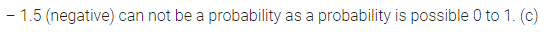Question 2.
If the probability of an event is p, then the probability of its complementary event will be
(a) p – 1
(b) p
(c) 1 – p
(d) $$1- \frac { 1 }{ p }$$
Solution:Question 3.
Out of one digit prime numbers, one selecting an even number is
(a) $$\\ \frac { 1 }{ 2 }$$
(b) $$\\ \frac { 1 }{ 4 }$$
(c) $$\\ \frac { 4 }{ 9 }$$
(d) $$\\ \frac { 2 }{ 5 }$$
Solution:Question 4.
Out of vowels, of the English alphabet, one letter is selected at random. The probability of selecting ‘e’ is
(a) $$\\ \frac { 1 }{ 26 }$$
(b) $$\\ \frac { 5 }{ 26 }$$
(c) $$\\ \frac { 1 }{ 4 }$$
(d) $$\\ \frac { 1 }{ 5 }$$
Solution: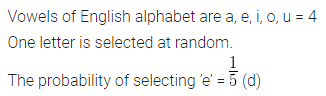Question 5.
When a die is thrown, the probability of getting an odd number less than 3 is
(a) $$\\ \frac { 1 }{ 6 }$$
(b) $$\\ \frac { 1 }{ 3 }$$
(c) $$\\ \frac { 1 }{ 2 }$$
(d) 0
Solution:Question 6.
A fair die is thrown once. The probability of getting an even prime number is
(a) $$\\ \frac { 1 }{ 6 }$$
(b) $$\\ \frac { 2 }{ 3 }$$
(c) $$\\ \frac { 1 }{ 3 }$$
(d) $$\\ \frac { 1 }{ 2 }$$
Solution: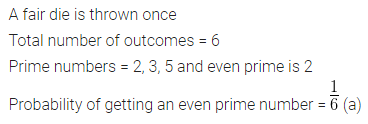Question 7.
A fair die is thrown once. The probability of getting a composite number is
(a) $$\\ \frac { 1 }{ 3 }$$
(b) $$\\ \frac { 1 }{ 6 }$$
(c) $$\\ \frac { 2 }{ 3 }$$
(d) 0
Solution: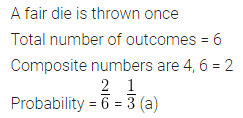Question 8.
If a fair dice is rolled once, then the probability of getting an even number or a number greater than 4 is
(a) $$\\ \frac { 1 }{ 2 }$$
(b) $$\\ \frac { 1 }{ 3 }$$
(c) $$\\ \frac { 5 }{ 6 }$$
(d) $$\\ \frac { 2 }{ 3 }$$
Solution:Question 9.
Rashmi has a die whose six faces show the letters as given below:If she throws the die once, then the probability of getting C is
(a) $$\\ \frac { 1 }{ 3 }$$
(b) $$\\ \frac { 1 }{ 4 }$$
(c) $$\\ \frac { 1 }{ 5 }$$
(d) $$\\ \frac { 1 }{ 6 }$$
Solution: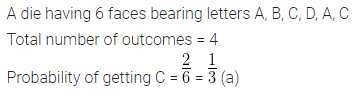Question 10.
If a letter is chosen at random from the letters of English alphabet, then the probability that it is a letter of the word ‘DELHI’ is
(a) $$\\ \frac { 1 }{ 5 }$$
(b) $$\\ \frac { 1 }{ 26 }$$
(c) $$\\ \frac { 5 }{ 26 }$$
(d) $$\\ \frac { 21 }{ 26 }$$
Solution: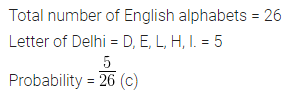Question 11.
A card is drawn from a well-shuffled pack of 52 playing cards. The event E is that the card drawn is not a face card. The number of outcomes favourable to the event E is
(a) 51
(b) 40
(c) 36
(d) 12
Solution: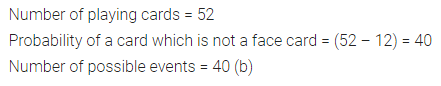Question 12.
A card is drawn from a deck of 52 cards. The event E is that card is not an ace of hearts. The number of outcomes favourable to E is
(a) 4
(b) 13
(c) 48
(d) 51
Solution: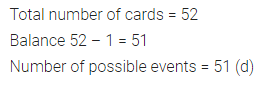Question 13.
If one card is drawn from a well-shuffled pack of 52 cards, the probability of getting an ace is
(a) $$\\ \frac { 1 }{ 52 }$$
(b) $$\\ \frac { 4 }{ 13 }$$
(c) $$\\ \frac { 2 }{ 13 }$$
(d) $$\\ \frac { 1 }{ 13 }$$
Solution:Question 14.
A card is selected at random from a well- shuffled deck of 52 cards. The probability of its being a face card is
(a) $$\\ \frac { 3 }{ 13 }$$
(b) $$\\ \frac { 4 }{ 13 }$$
(c) $$\\ \frac { 6 }{ 13 }$$
(d) $$\\ \frac { 9 }{ 13 }$$
Solution:Question 15.
A card is selected at random from a pack of 52 cards. The probability of its being a red face card is
(a) $$\\ \frac { 3 }{ 26 }$$
(b) $$\\ \frac { 3 }{ 13 }$$
(c) $$\\ \frac { 2 }{ 13 }$$
(d) $$\\ \frac { 1 }{ 2 }$$
Solution:Question 16.
If a card is drawn from a well-shuffled pack of 52 playing cards, then the probability of this card being a king or a jack is
(a) $$\\ \frac { 1 }{ 26 }$$
(b) $$\\ \frac { 1 }{ 13 }$$
(c) $$\\ \frac { 2 }{ 13 }$$
(d) $$\\ \frac { 4 }{ 13 }$$
Solution: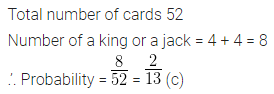Question 17.
The probability that a non-leap year selected at random has 53 Sundays is.
(a) $$\\ \frac { 1 }{ 365 }$$
(b) $$\\ \frac { 2 }{ 365 }$$
(c) $$\\ \frac { 2 }{ 7 }$$
(d) $$\\ \frac { 1 }{ 7 }$$
Solution:Question 18.
A bag contains 3 red balk, 5 white balls and 7 black balls. The probability that a ball drawn from the bag at random will be neither red nor black is
(a) $$\\ \frac { 1 }{ 5 }$$
(b) $$\\ \frac { 1 }{ 3 }$$
(c) $$\\ \frac { 7 }{ 15 }$$
(d) $$\\ \frac { 8 }{ 1 }$$
Solution:Question 19.
A bag contains 4 red balls and 5 green balls. One ball is drawn at random from the bag. The probability of getting either a red ball or a green ball is
(a) $$\\ \frac { 4 }{ 9 }$$
(b) $$\\ \frac { 5 }{ 9 }$$
(c) 0
(d) 1
Solution: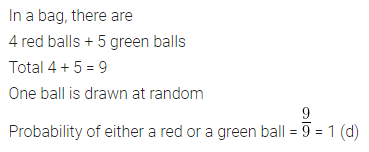Question 20.
A bag contains 5 red, 4 white and 3 black balls. If a. ball is drawn from the bag at random, then the probability of the ball being not black is
(a) $$\\ \frac { 5 }{ 12 }$$
(b) $$\\ \frac { 1 }{ 3 }$$
(c) $$\\ \frac { 3 }{ 4 }$$
(d) $$\\ \frac { 1 }{ 4 }$$
Solution:Question 21.
One ticket is drawn at random from a bag containing tickets numbered 1 to 40. The probability that the selected ticket has a number which is a multiple of 5 is
(a) $$\\ \frac { 1 }{ 5 }$$
(b) $$\\ \frac { 3 }{ 5 }$$
(c) $$\\ \frac { 4 }{ 5 }$$
(d) $$\\ \frac { 1 }{ 3 }$$
Solution:Question 22.
If a number is randomly chosen from the numbers 1, 2, 3, 4, …, 25, then the probability of the number to be prime is
(a) $$\\ \frac { 7 }{ 25 }$$
(b) $$\\ \frac { 9 }{ 25 }$$
(c) $$\\ \frac { 11 }{ 25 }$$
(d) $$\\ \frac { 13 }{ 25 }$$
Solution:Question 23.
A box contains 90 cards numbered 1 to 90. If one card is drawn from the box at random, then the probability that the number on the card is a perfect square is
(a) $$\\ \frac { 1 }{ 10 }$$
(b) $$\\ \frac { 9 }{ 100 }$$
(c) $$\\ \frac { 1 }{ 9 }$$
(d) $$\\ \frac { 1 }{ 100 }$$
Solution:Question 24.
If a (fair) coin is tossed twice, then the probability of getting two heads is
(a) $$\\ \frac { 1 }{ 4 }$$
(b) $$\\ \frac { 1 }{ 2 }$$
(c) $$\\ \frac { 3 }{ 4 }$$
(d) 0
Solution:Question 25.
If two coins are tossed simultaneously, then the probability of getting atleast one head is
(a) $$\\ \frac { 1 }{ 4 }$$
(b) $$\\ \frac { 1 }{ 2 }$$
(c) $$\\ \frac { 3 }{ 4 }$$
(d) 1
Solution:Question 26.
Lakshmi tosses two coins simultaneously. The probability that she gets almost one head
(a) 1
(b) $$\\ \frac { 3 }{ 4 }$$
(c) $$\\ \frac { 1 }{ 2 }$$
(d) $$\\ \frac { 1 }{ 7 }$$
Solution:Question 27.
The probability of getting a bad egg in a lot of 400 eggs is 0.035. The number of bad eggs in the lot is
(a) 7
(b) 14
(c) 21
(d) 28
Solution: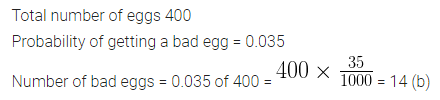Question 28.
A girl calculates that the probability of her winning the first prize in a lottery is 0.08. If 6000 tickets are sold, how many tickets she has bought?
(a) 40
(b) 240
(c) 480
(d) 750
Solution: